1. /
2. CBSE
3. /
4. Class 08
5. /
6. Mathematics
7. /
8. NCERT Solutions for Class...

# NCERT Solutions for Class 8 Maths Exercise 16.2### myCBSEguide App

Download the app to get CBSE Sample Papers 2023-24, NCERT Solutions (Revised), Most Important Questions, Previous Year Question Bank, Mock Tests, and Detailed Notes.

NCERT solutions for Class 8 Maths Playing with Numbers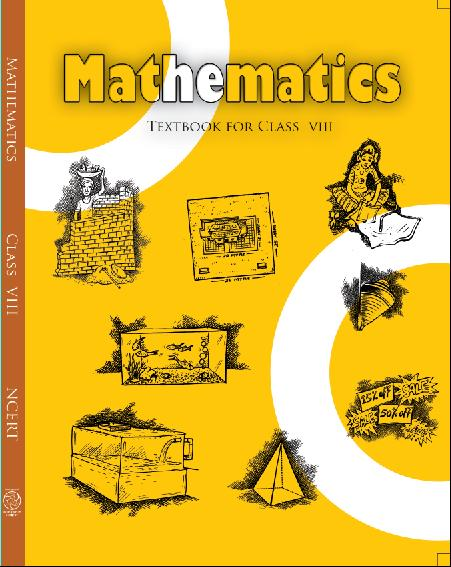## NCERT Solutions for Class 8 Maths Playing with Numbers

###### 1. If 21y5 is a multiple of 9, where y is a digit, what is the value of y?

Ans. Since 21y5 is a multiple of 9.

Therefore according to the divisibility rule of 9, the sum of all the digits should be a multiple of 9.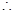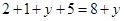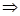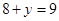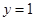Since 21y5 is a multiple of 9.

###### 2. If 31z5 is a multiple of 9, where z is a digit, what is the value of z? You will find that there are two answers for the last problem. Why is this so?

Ans.  Since 31z5 is a multiple of 9.

Therefore according to the divisibility rule of 9, the sum of all the digits should be a multiple of 9.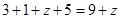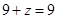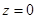If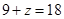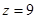Hence 0 and 9 are two possible answers.

NCERT Solutions for Class 8 Maths Exercise 16.2

###### 3. If 24x is a multiple of 3, where x is a digit, what is the value of x?

(Since 24x is a multiple of 3, its sum of digits 6 + x is a multiple of 3; so 6 + x is one of these numbers: 0, 3, 6, 9, 12, 15, 18, … .But since x is a digit, it can only be that
6 + x = 6 or 9 or 12 or 15. Therefore, x = 0 or 3 or 6 or 9. Thus, x can have any of (four different values.)

Ans.  Since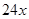is a multiple of 3.

Therefore according to the divisibility rule of 3, the sum of all the digits should be a multiple of 3.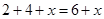Since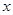is a digit.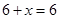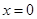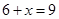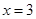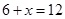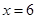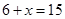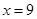Thus,can have any of four different values.

NCERT Solutions for Class 8 Maths Exercise 16.2

###### 4.     If 31z5 is a multiple of 3, where z is a digit, what might be the values of z?

Ans. Since 31z5 is a multiple of 3.

Therefore according to the divisibility rule of 3, the sum of all the digits should be a multiple of 3.

Since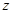is a digit.If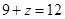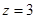If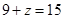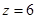IfHence 0, 3, 6 and 9 are four possible answers.

## NCERT Solutions for Class 8 Maths Exercise 16.2

NCERT Solutions Class 8 Mathematics PDF (Download) Free from myCBSEguide app and myCBSEguide website. Ncert solution class 8 Mathematics includes text book solutions from Class 8 Maths Book . NCERT Solutions for CBSE Class 8 Maths have total 16 chapters. 8 Maths NCERT Solutions in PDF for free Download on our website. Ncert class 8 solutions PDF and Maths ncert class 8 PDF solutions with latest modifications and as per the latest CBSE syllabus are only available in myCBSEguide.

## CBSE app for Class 8

To download NCERT Solutions for class 8 Social Science, Computer Science, Home Science,Hindi ,English, Maths Science do check myCBSEguide app or website. myCBSEguide provides sample papers with solution, test papers for chapter-wise practice, NCERT solutions, NCERT Exemplar solutions, quick revision notes for ready reference, CBSE guess papers and CBSE important question papers. Sample Paper all are made available through the best app for CBSE students and myCBSEguide website.### Test Generator

Create question paper PDF and online tests with your own name & logo in minutes.### myCBSEguide

Question Bank, Mock Tests, Exam Papers, NCERT Solutions, Sample Papers, Notes

1. Hello

2. vere nice